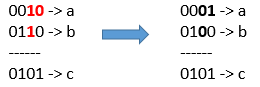## 1318. Minimum Flips to Make a OR b Equal to c

Given 3 positives numbers `a`, `b` and `c`. Return the minimum flips required in some bits of `a` and `b` to make ( `a` OR `b` == `c` ). (bitwise OR operation).
Flip operation consists of change any single bit 1 to 0 or change the bit 0 to 1 in their binary representation.

Example 1:```Input: a = 2, b = 6, c = 5
Output: 3
Explanation: After flips a = 1 , b = 4 , c = 5 such that (`a` OR `b` == `c`)```

Example 2:

```Input: a = 4, b = 2, c = 7
Output: 1
```

Example 3:

```Input: a = 1, b = 2, c = 3
Output: 0
```

Constraints:

• `1 <= a <= 10^9`
• `1 <= b <= 10^9`
• `1 <= c <= 10^9`

## Rust Solution

``````struct Solution;

impl Solution {
fn min_flips(a: i32, b: i32, c: i32) -> i32 {
let mut res = 0;
for i in 0..32 {
let aa = (a >> i) & 1;
let bb = (b >> i) & 1;
let cc = (c >> i) & 1;
if cc == 0 {
if aa == 1 {
res += 1;
}
if bb == 1 {
res += 1;
}
} else {
if aa == 0 && bb == 0 {
res += 1;
}
}
}
res
}
}

#[test]
fn test() {
let a = 2;
let b = 6;
let c = 5;
let res = 3;
assert_eq!(Solution::min_flips(a, b, c), res);
let a = 4;
let b = 2;
let c = 7;
let res = 1;
assert_eq!(Solution::min_flips(a, b, c), res);
let a = 1;
let b = 2;
let c = 3;
let res = 0;
assert_eq!(Solution::min_flips(a, b, c), res);
let a = 8;
let b = 3;
let c = 5;
let res = 3;
assert_eq!(Solution::min_flips(a, b, c), res);
}
``````

Having problems with this solution? Click here to submit an issue on github.